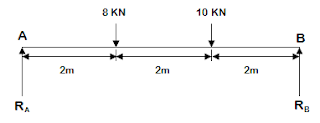# How to find supports reaction | Solved problems of structural analysis

## Here, you will know calculating reactions at support of structure as given in problems:

### A simply supported beam of 6 m span has two concentrated load of 8 KN and 10 KN at 2 m and 4 m from left hand support. Find the reaction at two supports.

Sol. Draw a figure of beam as given in question:Considering the beam to be in equilibrium:

ΣfY = 0 upward force taken as +ve and downward taken as –ve.

Then, RA + RB - 8 - 10 = 0

Or, RA + RB = 18 ….. (1)

Taking moment et end A,

ΣMA = 0 Clockwise moment taken as +ve and anti clockwise taken as –ve.

Then, (-RB x 6) + (10 x 4) + (8 x 2) = 0

Or, -6 RB + 40 + 16 = 0

RB = 56/6 = 9.33 ….. (2)

Now, Put value of RB in eq. (1)

RA + 9.33 = 18

Or, RA = 18 - 9.33 = 8.67 KN

So, RA = 8.67 KN and RB = 9.33 KN are calculated two reaction force at supports.

### A beam AB of 9 m span is simply supported at the ends. The beam carries point load of 2 KN upwards at 2 m from A and uniformly distributed load of 1000 N/m downwards on a length of 6 m from B. determine the supports reaction.

Sol. Draw a figure of beam as given in question:

Considering the beam to be in equilibrium:

ΣfY = 0 upward force taken as +ve and downward taken as –ve.

1000 x 6 = 6000 N = 6 KN, at 6/2 = 3 m

Then, RA + RB + 2 - 6 = 0

Or, RA + RB = 4 ….. (1)

Taking moment at end A,

ΣMA = 0 Clockwise moment taken as +ve and anti clockwise taken as –ve.

Then, (-RB x 9) + (6 x 6) + (-2 x 2) = 0

Or, -9 RB + 36 - 4 = 0

RB = 32/9 = 3.55 ….. (2)

Now, Put value of RB in eq. (1)

RA + 3.55 = 4

Or, RA = 4 - 3.55 = 0.45 KN

So, RA = 0.45 KN and RB = 3.55 KN are calculated two reaction force at supports.

Related Topics :

Civil Engineering Objective (MCQ) Book pdf by R S Khurmi and JK Gupta

Can Home Makes All Its Own Energy | Zero energy building, Green building?